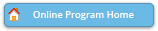#### Abstract Details

 Activity Number: 379 Type: Contributed Date/Time: Tuesday, August 2, 2016 : 10:30 AM to 12:20 PM Sponsor: Section on Statistics in Imaging Abstract #320058 Title: Estimating the Coefficients of a Linear Differential Operator to Model Functional Data Author(s): Maria Ivette Barraza-Rios* and Joan G. Staniswalis Companies: The University of Texas at El Paso and The University of Texas at El Paso Keywords: principal differential analysis ; functional data ; low-dimensional approximations Abstract: Principal Differential Analysis (PDA; Ramsay, 1996) is used to obtain low-dimensional representations of functional data, where each observation may be represented as a curve. PDA seeks to identify a Linear Differential Operator (LDO) denoted by L that satisfies as closely as possible that Lf=0 for each functional observation f. A theorem from analysis establishes that the coefficients of the LDO are in the Sobolev space, and thus can be approximated by B-splines. Current PDA software used to estimate the LDO assumes that the leading coefficient is 1. We present a method that eliminates this restriction, and ensures that the coefficients of the LDO are in the Sobolev space, and that their approximation by B-splines is mathematically valid. The proposed method is inspired by results in linear regression (Frees, 1991 and Wu, 1986) that show that the weighted average of pairwise slopes between data points is equivalent to the least squares estimator of the regression line slope. By analyzing data, the proposed method is compared with pda.fd (R library fda).

Authors who are presenting talks have a * after their name.